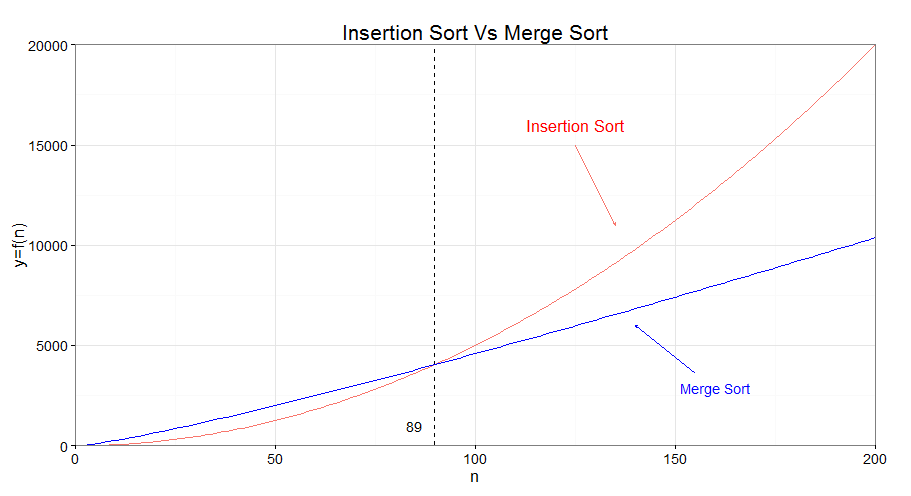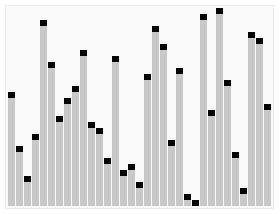# Algorithm - Big O Notation

The big-Oh notation is a vocabulary for the design and analysis of algorithms.

See:

## Examples

• $6n log_2 n + 6$ is just $n log n$ .
• Terminology: running time is O(n log n) [“big-Oh” of n log n] where n = input size (e.g. length of input array).

### One Loop

Does array A contain the integer t?

for i=1 to n do
if A[i] == t then
Return TRUE
Return FALSE


The running time of this algorithm is linear in the input length n: O(n).

In the worse case, this code will do an unsuccessful search.

Whatever the constant (2, 3, 4…) is due some initial and final operation, it gets conveniently suppressed by the Big O notation.

### Two Loops

Given A, B (arrays of length n) and t (an integer). [Does A or B contain t?]

for i=1 to n do
if A[i] == t then
Return TRUE
for i=1 to n do
if B[i] == t then
Return TRUE
Return FALSE


The running time will be roughly twice as many operations. as the previous piece of code : $2n$

But the coefficient two, being a constant independent of the input length n, is going to get suppressed once we passed a big O notation.

The running time is $O(n)$

### Two Nested Loops

Do arrays A, B have a number in common? Given arrays A, B of length n.

for i=1 to n do
for j=1 to n do
if A[i] == B[j] then
Return TRUE
Return FALSE


The running time is $O(n^2)$

This a quadratic time algorithm. because the running time is quadratic in the input length n.

### Two Nested Loops ()

Does array A have duplicate entries? Given arrays A of length n.

for i=1 to n do
for j=i+1 to n do
if A[i] == A[j] then
Return TRUE
Return FALSE


There's n choose 2 such choices of distinct i and j.

Again, suppressing lower-order terms and the constant factor, we still get a quadratic dependence on the length of the input array A.

The running time is $O(n^2)$

Discover MoreAlgorithm - Asymptotic Analysis

Asymptotic analysis provides basic vocabulary for discussing the design and analysis in algorithms. This is the language by which every serious computer programmer and computer scientist discusses...Performance - Constant Time - O(1)

A algorithm executes in constant time if no matter how large N is, it will always execute with the same latency (time). In Big O notation, it performs as A hashmap performs the IO operation get and...